• 分享知识要点：lubridate包拆解时间 | POSIXlt利用决策树分类，利用随机森林预测利用对数进行fit，和exp函数还原训练集来自Kaggle华盛顿自行车共享计划中的自行车租赁数据分析共享自行车天气、时间等关系。数据...
编辑推荐:来源于cnblogs，介绍了利用决策树分类，利用随机森林预测，利用对数进行fit，和exp函数还原等。分享知识要点：lubridate包拆解时间 | POSIXlt利用决策树分类，利用随机森林预测利用对数进行fit，和exp函数还原训练集来自Kaggle华盛顿自行车共享计划中的自行车租赁数据，分析共享自行车与天气、时间等关系。数据集共11个变量，10000多行数据。https://www.kaggle.com/c/bike-sharing-demand首先看一下官方给出的数据，一共两个表格，都是2011-2012年的数据，区别是Test文件是每个月的日期都是全的，但是没有注册用户和随意用户。而Train文件是每个月只有1-20天，但有两类用户的数量。求解：补全Train文件里21-30号的用户数量。评价标准是预测与真实数量的比较。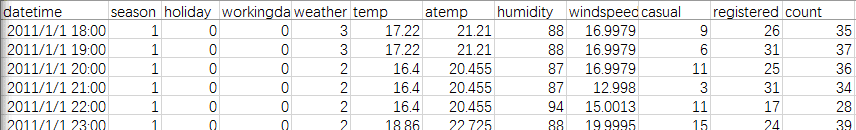1.png首先加载文件和包library (lubridate)library (randomForest)library (readr)setwd ("E:")data <-read_csv ("train.csv")head (data)这里我就遇到坑了，用r语言缺省的read.csv死活读不出来正确的文件格式，换成xlsx更惨，所有时间都变成43045这样的怪数字。本来之前试过as.Date可以正确转换，但这次因为有时分秒，就只能用时间戳，但结果也不行。最后是下载了"readr"包，用read_csv语句，顺利解读。因为test比train日期完整，但缺少用户数，所以要把train和test合并。test$registered=0test$casual=0test$count=0data<-rbind(train,test)摘取时间：可以用时间戳，这里的时间比较简单，就是小时数，所以也可以直接截字符串。data$hour1<-substr(data$datetime,12,13)table(data$hour1)统计一下每个小时的使用总数，是这样（为什么介么整齐）：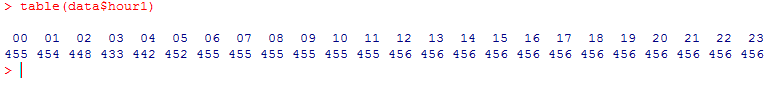6-hour1.png接下来是运用箱线图，看一下使用者和时间，周几这些的关系。为什么用箱线图而不用hist直方图，因为箱线图有离散点表达，下面也因此运用对数求fit从图中可以看出，在时间方面，注册用户和非注册用户的使用时间有很大不同。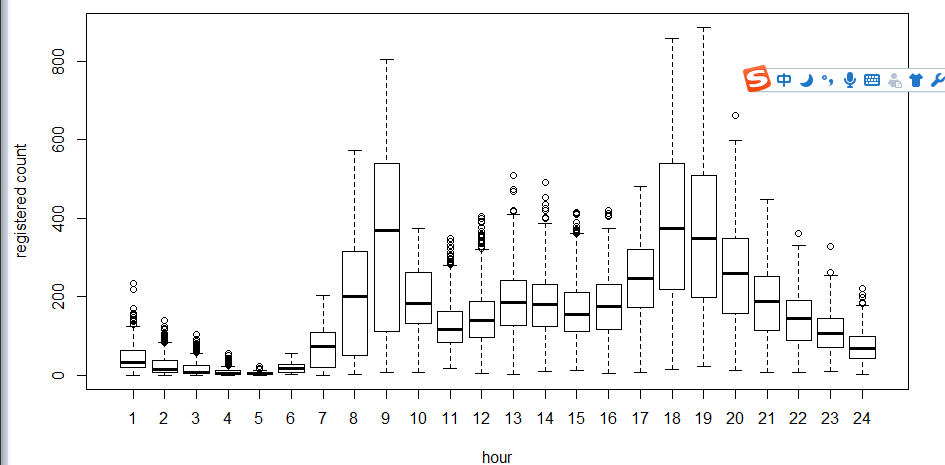5-hour-regestered.png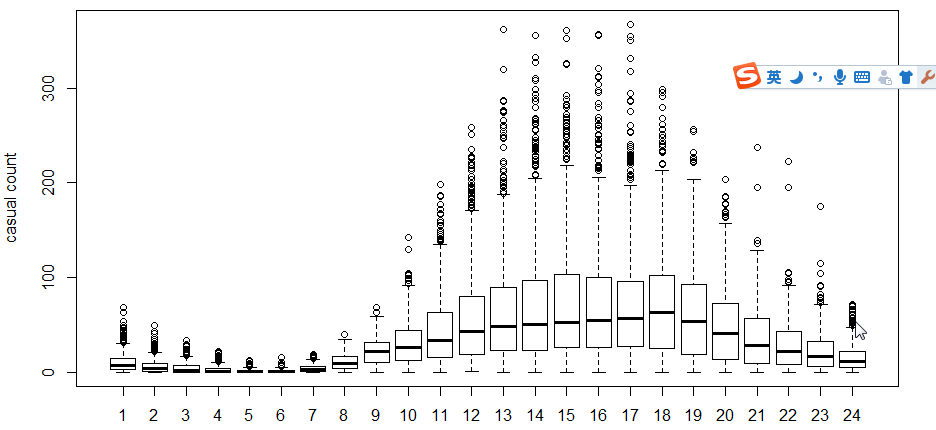5-hour-casual.png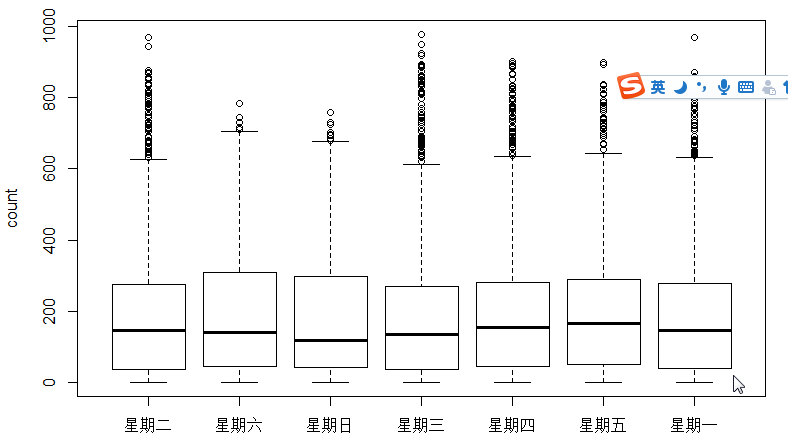4-boxplot-day.png接下来用相关系数cor检验用户，温度，体感温度，湿度，风速的关系。相关系数：变量之间的线性关联度量，检验不同数据的相关程度。取值范围[-1，1]，越接近0越不相关。从运算结果可以看出，使用人群与风速呈负相关，比温度影响还大。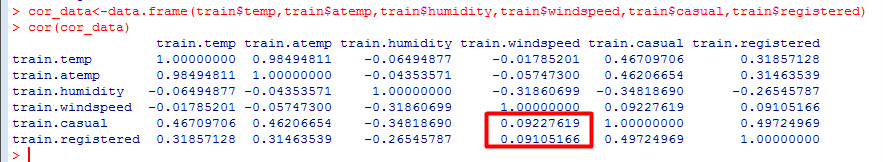cor.png接下来就是将时间等因素用决策树分类，然后用随机森林来预测。随机森林和决策树的算法。听起来很高大上，其实现在也很常用了，所以一定要学会。决策树模型是 一种简单易用的非参数分类器。它不需要对数据有任何的先验假设，计算速度较快，结果容易解释，而且稳健性强，不怕噪声数据和缺失数据。决策树模型的基本计 算步骤如下：先从n个自变量中挑选一个，寻找最佳分割点，将数据划分为两组。针对分组后数据，将上述步骤重复下去，直到满足某种条件。在决策树建模中需要解决的重要问题有三个：如何选择自变量如何选择分割点确定停止划分的条件做出注册用户和小时的决策树，train$hour1<-as.integer(train$hour1)d<-rpart (registered~hour1,data=train)rpart .plot(d) >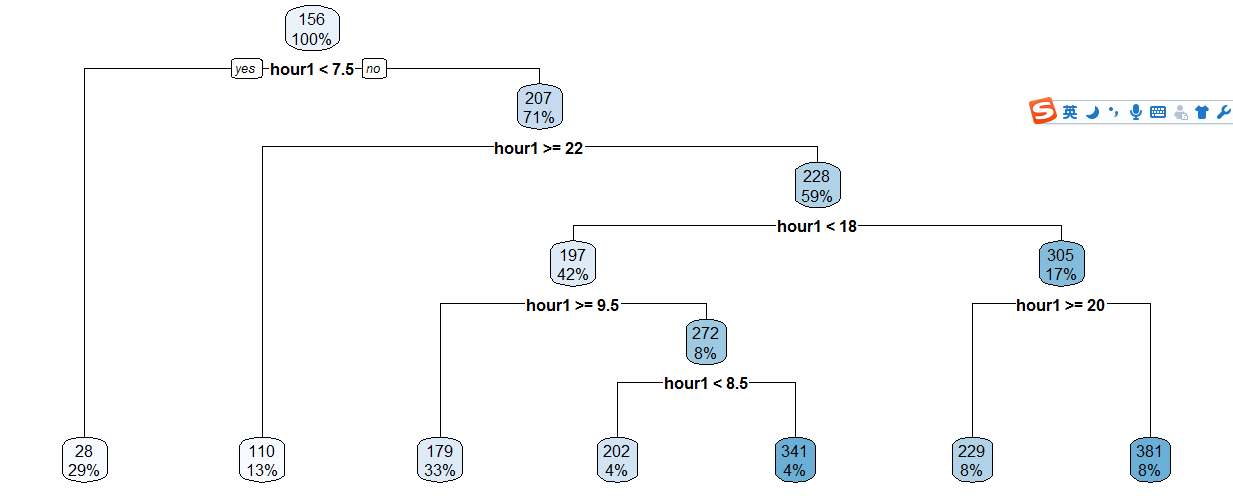3-raprt-hour1.png然后就是根据决策树的结果手动分类，所以还满占代码的...train$hour1<-as.integer(train$hour1)data$dp_reg=0data$dp_reg[data$hour1 <7.5]=1data$dp_reg[data$hour1> =22]=2data$dp_reg[data$hour1 >=9.5 & data$hour1<18]=3data$dp_reg[data$hour1> =7.5 & data$hour1<18]=4data$dp_reg[data$hour1> =8.5 & data$hour1<18]=5data$dp_reg[data$hour1> =20 & data$hour1<20]=6data$dp_reg[data$hour1> =18 & data$hour1<20]=7同理，做出 （小时 | 温度） X （注册 | 随意用户） 等决策树，继续手动分类....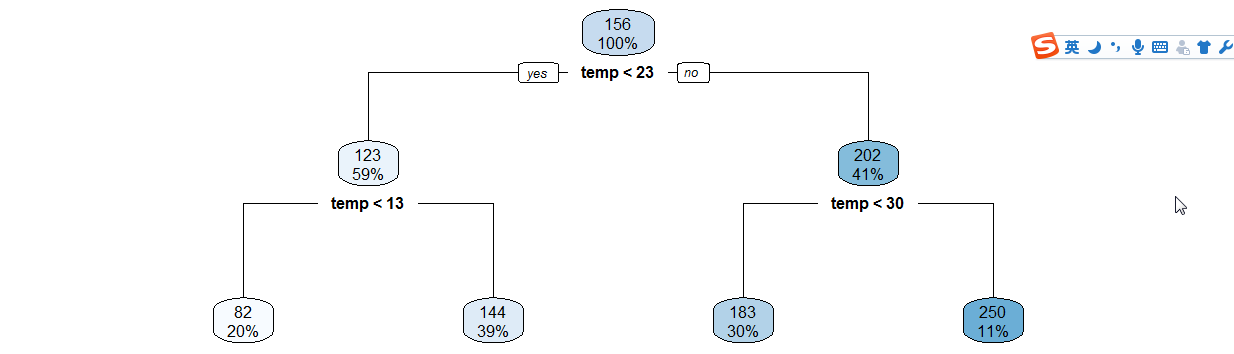3-raprt-temp.png年份月份，周末假日等手动分类data$year_part=0data$month <-month(data$datatime)data$year_part [data$year=='2011']=1data$year_part [data$year=='2011' & data$month>3]= 2data$year_part[data$year=='2011' & data $month>6]= 3data$year_part[data$year=='2011' & data$month>9]= 4data$day_type=""data$day _type [data$holiday ==0 & data$workingday==0]="weekend"data$day_type[data$holiday==1] ="holiday"data$day_type[data$holiday ==0 & data$workingday==1]="working day"data$weekend=0data$weekend [data$day= ="Sunday"|data$day==" Saturday "] =1接下来用随机森林语句预测在机器学习中，随机森林是一个包含多个决策树的分类器， 并且其输出的类别是由个别树输出的类别的众数而定。随机森林中的子树的每一个分裂过程并未用到所有的待选特征，而是从所有的待选特征中随机选取一定的特征，再在其中选取最优的特征。这样决策树都能够彼此不同，提升系统的多样性，从而提升分类性能。ntree指定随机森林所包含的决策树数目，默认为500，通常在性能允许的情况下越大越好；mtry指定节点中用于二叉树的变量个数，默认情况下数据集变量个数的二次方根（分类模型）或三分之一（预测模型）。一般是需要进行人为的逐次挑选，确定最佳的m值―摘自datacruiser笔记。这里我主要学习，所以虽然有10000多数据集，但也只定了500。就这500我的小电脑也跑了半天。train<-dataset.seed (1234)train$logreg <-log(train$registered+1)test$logcas <-log(train$casual+1)fit1 <-randomForest (logreg~hour1+ workingday+ day + holiday + day_ type+ temp_reg+ humidity+ atemp + windspeed + season+ weather+ dp_ reg+weekend + year+year _part ,train ,importance =TRUE , ntree = 250)pred1 <-predict (fit1,train)train$logreg <-pred1这里不知道怎么回事，我的day和day_part加进去就报错，只有删掉这两个变量计算，还要研究修补。然后用exp函数还原train$registered<-exp(train$logreg)-1train$casual<-exp(train$logcas)-1train$count<-test$casual+train$registered最后把20日后的日期截出来，写入新的csv文件上传。train2<-train[as.integer(day(data$datetime))> = 20 ,]submit_final <-data.frame (datetime= test$datetime ,count= test$count)write.csv(submit _final,"submit_ final.csv", row .names = F)
展开全文• 分享知识要点：lubridate包拆解时间 | POSIXlt利用决策树分类，利用随机森林预测利用对数进行fit，和exp函数还原训练集来自Kaggle华盛顿自行车共享计划中的自行车租赁数据分析共享自行车天气、时间等关系。数据...
分享知识要点：lubridate包拆解时间 | POSIXlt利用决策树分类，利用随机森林预测利用对数进行fit，和exp函数还原训练集来自Kaggle华盛顿自行车共享计划中的自行车租赁数据，分析共享自行车与天气、时间等关系。数据集共11个变量，10000多行数据。https://www.kaggle.com/c/bike-sharing-demand首先看一下官方给出的数据，一共两个表格，都是2011-2012年的数据，区别是Test文件是每个月的日期都是全的，但是没有注册用户和随意用户。而Train文件是每个月只有1-20天，但有两类用户的数量。求解：补全Train文件里21-30号的用户数量。评价标准是预测与真实数量的比较。1.png首先加载文件和包library(lubridate)library(randomForest)library(readr)setwd("E:")data<-read_csv("train.csv")head(data)这里我就遇到坑了，用r语言缺省的read.csv死活读不出来正确的文件格式，换成xlsx更惨，所有时间都变成43045这样的怪数字。本来之前试过as.Date可以正确转换，但这次因为有时分秒，就只能用时间戳，但结果也不行。最后是下载了"readr"包，用read_csv语句，顺利解读。因为test比train日期完整，但缺少用户数，所以要把train和test合并。test$registered=0test$casual=0test$count=0data<-rbind(train,test)摘取时间：可以用时间戳，这里的时间比较简单，就是小时数，所以也可以直接截字符串。data$hour1<-substr(data$datetime,12,13)table(data$hour1)统计一下每个小时的使用总数，是这样（为什么介么整齐）：6-hour1.png接下来是运用箱线图，看一下使用者和时间，周几这些的关系。为什么用箱线图而不用hist直方图，因为箱线图有离散点表达，下面也因此运用对数求fit从图中可以看出，在时间方面，注册用户和非注册用户的使用时间有很大不同。5-hour-regestered.png5-hour-casual.png4-boxplot-day.png接下来用相关系数cor检验用户，温度，体感温度，湿度，风速的关系。相关系数：变量之间的线性关联度量，检验不同数据的相关程度。取值范围[-1，1]，越接近0越不相关。从运算结果可以看出，使用人群与风速呈负相关，比温度影响还大。cor.png接下来就是将时间等因素用决策树分类，然后用随机森林来预测。随机森林和决策树的算法。听起来很高大上，其实现在也很常用了，所以一定要学会。决策树模型是 一种简单易用的非参数分类器。它不需要对数据有任何的先验假设，计算速度较快，结果容易解释，而且稳健性强，不怕噪声数据和缺失数据。决策树模型的基本计 算步骤如下：先从n个自变量中挑选一个，寻找最佳分割点，将数据划分为两组。针对分组后数据，将上述步骤重复下去，直到满足某种条件。在决策树建模中需要解决的重要问题有三个：如何选择自变量如何选择分割点确定停止划分的条件做出注册用户和小时的决策树，train$hour1<-as.integer(train$hour1)d<-rpart(registered~hour1,data=train)rpart.plot(d)3-raprt-hour1.png然后就是根据决策树的结果手动分类，所以还满占代码的...train$hour1<-as.integer(train$hour1)data$dp_reg=0data$dp_reg[data$hour1<7.5]=1data$dp_reg[data$hour1>=22]=2data$dp_reg[data$hour1>=9.5 & data$hour1<18]=3data$dp_reg[data$hour1>=7.5 & data$hour1<18]=4data$dp_reg[data$hour1>=8.5 & data$hour1<18]=5data$dp_reg[data$hour1>=20 & data$hour1<20]=6data$dp_reg[data$hour1>=18 & data$hour1<20]=7同理，做出 （小时 | 温度） X （注册 | 随意用户） 等决策树，继续手动分类....3-raprt-temp.png年份月份，周末假日等手动分类data$year_part=0data$month<-month(data$datatime)data$year_part[data$year=='2011']=1data$year_part[data$year=='2011' & data$month>3]=2data$year_part[data$year=='2011' & data$month>6]=3data$year_part[data$year=='2011' & data$month>9]=4data$day_type=""data$day_type[data$holiday==0 & data$workingday==0]="weekend"data$day_type[data$holiday==1]="holiday"data$day_type[data$holiday==0 & data$workingday==1]="working day"data$weekend=0data$weekend[data$day=="Sunday"|data$day=="Saturday"]=1接下来用随机森林语句预测在机器学习中，随机森林是一个包含多个决策树的分类器， 并且其输出的类别是由个别树输出的类别的众数而定。随机森林中的子树的每一个分裂过程并未用到所有的待选特征，而是从所有的待选特征中随机选取一定的特征，再在其中选取最优的特征。这样决策树都能够彼此不同，提升系统的多样性，从而提升分类性能。ntree指定随机森林所包含的决策树数目，默认为500，通常在性能允许的情况下越大越好；mtry指定节点中用于二叉树的变量个数，默认情况下数据集变量个数的二次方根（分类模型）或三分之一（预测模型）。一般是需要进行人为的逐次挑选，确定最佳的m值—摘自datacruiser笔记。这里我主要学习，所以虽然有10000多数据集，但也只定了500。就这500我的小电脑也跑了半天。train<-dataset.seed(1234)train$logreg<-log(train$registered+1)test$logcas<-log(train$casual+1)fit1<-randomForest(logreg~hour1+workingday+day+holiday+day_type+temp_reg+humidity+atemp+windspeed+season+weather+dp_reg+weekend+year+year_part,train,importance=TRUE,ntree=250)pred1<-predict(fit1,train)train$logreg<-pred1这里不知道怎么回事，我的day和day_part加进去就报错，只有删掉这两个变量计算，还要研究修补。然后用exp函数还原train$registered<-exp(train$logreg)-1train$casual<-exp(train$logcas)-1train$count<-test$casual+train$registered最后把20日后的日期截出来，写入新的csv文件上传。train2<-train[as.integer(day(data$datetime))>=20,]submit_final<-data.frame(datetime=test$datetime,count=test$count)write.csv(submit_final,"submit_final.csv",row.names=F)大功告成！github代码加群原来的示例是炼数成金网站的kaggle课程第二节，基本按照视频的思路。因为课程没有源代码，所以要自己修补运行完整。历时两三天总算把这个功课做完了。下面要修正的有：好好理解三个知识点（lubridate包/POSIXlt，log线性，决策树和随机森林）；用WOE和IV代替cor函数分析相关关系；用其他图形展现的手段分析随机树变量重新测试学习过程中遇到什么问题或者想获取学习资源的话，欢迎加入学习交流群626062078，我们一起学Python！完成了一个"浩大完整”的数据分析，还是很有成就感的！
展开全文• 无意中发现了一个巨牛的人工智能教程，忍不住分享...Python的功能不可以说不大，在金融数据分析里面有着很方便的应用。 1.数据获取 pandas包中有自带的数据获取接口，详细的大家可以去其官网上找，是io.data下的...
 无意中发现了一个巨牛的人工智能教程，忍不住分享一下给大家。教程不仅是零基础，通俗易懂，而且非常风趣幽默，像看小说一样！觉得太牛了，所以分享给大家。教程链接：https://www.cbedai.net/qtlyx

Python的功能不可以说不大，在金融数据分析里面有着很方便的应用。

1.数据获取

pandas包中有自带的数据获取接口，详细的大家可以去其官网上找，是io.data下的DataReader方法。

import numpy as np
import pandas as pd
import pandas.io.data as web
import math
#从雅虎财经获取DAX指数的数据
DAX = web.DataReader(name='^GDAXI', data_source='yahoo',start = '2000-1-1')
#查看一下数据的一些信息 上面这一方法返回的是一个pandas dataframe的数据结构
print DAX.info()
#绘制收盘价的曲线
DAX['Close'].plot(figsize=(8,5))

我们获得的数据是dataframe的结构，毕竟是pandas的接口的嘛。然后我们绘制一下收盘价曲线。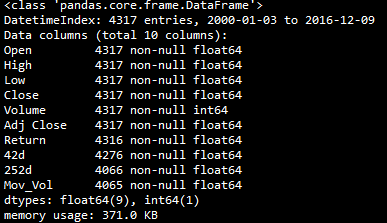这个是我们获取的数据的信息。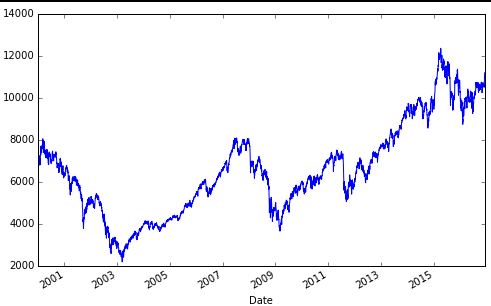绘制出来的收盘价曲线是这样的。

2.简单的数据处理

有了股票价格，我们就计算一下每天的涨跌幅度，换句话说，就是每天的收益率，以及股价的移动平均和股价的波动率。

#计算每日的涨跌幅
DAX['Return'] = np.log(DAX['Close']/DAX['Close'].shift(1))
print DAX[['Close','Return']].tail()
#将收盘价与每日涨跌幅度放在一张图上
DAX[['Close','Return']].plot(subplots = True,style = 'b',figsize=(8,5))
#42与252个交易日为窗口取移动平均
DAX['42d']=pd.rolling_mean(DAX['Close'],window=42)
DAX['252d']=pd.rolling_mean(DAX['Close'],window=252)
#绘制MA与收盘价
DAX[['Close','42d','252d']].plot(figsize=(8,5))
#计算波动率，然后根据均方根法则进行年化
DAX['Mov_Vol']=pd.rolling_std(DAX['Return'],window = 252)*math.sqrt(252)
DAX[['Close','Mov_Vol','Return']].plot(subplots = True, style = 'b',figsize = (8,7))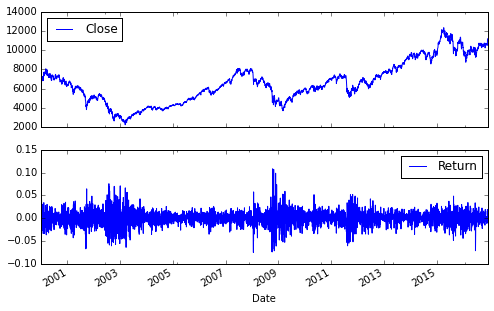我们可以掌握这种subplots的绘图方法，把几张趋势图片放在一起。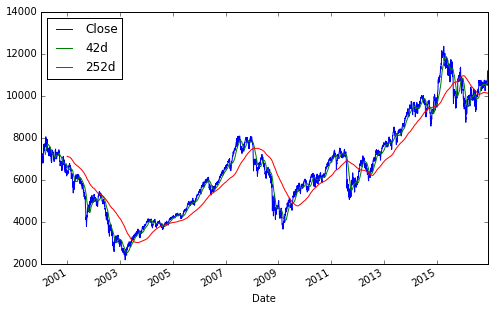这是移动平均线的图片，subplots的属性为false，那么就是叠加在一起绘制。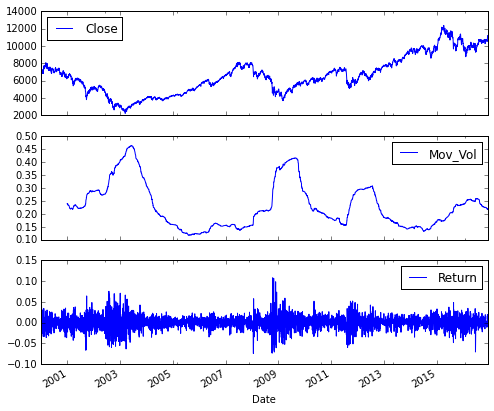这是市场的波动率和股市的关系。和FRM中提到的一样，在市场低迷，或者说，金融危机的时候，市场的波动率急剧增加。于是，就有了恐慌指数这个东西，也就是Vix，其实就是市场的波动率指数。


展开全文大数据 股票 量化投资
• 3.1 Pandas 简介;3.1 Pandas 简介导入Pandas包;3.2 序列;3.2.1 序列创建及访问;3.2.1 序列的创建及访问;3.2.2 序列属性;...3.2.3 序列方法空值处理方法 ;3.2.4 序列切片;3.2.4 序列聚合运算;3.3 数据框;3.3.1 数
• 第2部分介绍了金融分析和应用程序开发中重要的Python库、技术和方法，其内容涵盖了Python的数据类型和结构、用matplotlib进行数据可视化、金融时间序列数据处理、高性能输入/输出操作、高性能的Python技术和库、金融...
• 【入门基础+轻实战演示】【讲授方式轻松幽默、有趣不枯燥、案例实操结合，相关课程差异化】利用python进行数据处理分析，并结合大量具体的例子，对每个知识进行实战讲解，本课程通过大量练习和案例对各个知识...编程语言
• 利用对数进行fit，和exp函数还原训练集来自Kaggle华盛顿自行车共享计划中的自行车租赁数据分析共享自行车天气、时间等关系。数据集共11个变量，10000多行数据。 https://www.kaggle.com/c/bike-sharing-demand...
• 分享知识要点：lubridate包拆解时间 | POSIXlt利用决策树分类，利用随机森林预测利用对数进行fit，和exp函数还原训练集来自Kaggle华盛顿自行车共享计划中的自行车租赁数据分析共享自行车天气、时间等关系。数据...
分享知识要点：lubridate包拆解时间 | POSIXlt利用决策树分类，利用随机森林预测利用对数进行fit，和exp函数还原训练集来自Kaggle华盛顿自行车共享计划中的自行车租赁数据，分析共享自行车与天气、时间等关系。数据集共11个变量，10000多行数据。https://www.kaggle.com/c/bike-sharing-demand首先看一下官方给出的数据，一共两个表格，都是2011-2012年的数据，区别是Test文件是每个月的日期都是全的，但是没有注册用户和随意用户。而Train文件是每个月只有1-20天，但有两类用户的数量。求解：补全Train文件里21-30号的用户数量。评价标准是预测与真实数量的比较。1.png首先加载文件和包library(lubridate)library(randomForest)library(readr)setwd("E:")data<-read_csv("train.csv")head(data)这里我就遇到坑了，用r语言缺省的read.csv死活读不出来正确的文件格式，换成xlsx更惨，所有时间都变成43045这样的怪数字。本来之前试过as.Date可以正确转换，但这次因为有时分秒，就只能用时间戳，但结果也不行。最后是下载了"readr"包，用read_csv语句，顺利解读。因为test比train日期完整，但缺少用户数，所以要把train和test合并。test$registered=0test$casual=0test$count=0data<-rbind(train,test)摘取时间：可以用时间戳，这里的时间比较简单，就是小时数，所以也可以直接截字符串。data$hour1<-substr(data$datetime,12,13)table(data$hour1)统计一下每个小时的使用总数，是这样（为什么介么整齐）：6-hour1.png接下来是运用箱线图，看一下使用者和时间，周几这些的关系。为什么用箱线图而不用hist直方图，因为箱线图有离散点表达，下面也因此运用对数求fit从图中可以看出，在时间方面，注册用户和非注册用户的使用时间有很大不同。5-hour-regestered.png5-hour-casual.png4-boxplot-day.png接下来用相关系数cor检验用户，温度，体感温度，湿度，风速的关系。相关系数：变量之间的线性关联度量，检验不同数据的相关程度。取值范围[-1，1]，越接近0越不相关。从运算结果可以看出，使用人群与风速呈负相关，比温度影响还大。cor.png接下来就是将时间等因素用决策树分类，然后用随机森林来预测。随机森林和决策树的算法。听起来很高大上，其实现在也很常用了，所以一定要学会。决策树模型是 一种简单易用的非参数分类器。它不需要对数据有任何的先验假设，计算速度较快，结果容易解释，而且稳健性强，不怕噪声数据和缺失数据。决策树模型的基本计 算步骤如下：先从n个自变量中挑选一个，寻找最佳分割点，将数据划分为两组。针对分组后数据，将上述步骤重复下去，直到满足某种条件。在决策树建模中需要解决的重要问题有三个：如何选择自变量如何选择分割点确定停止划分的条件做出注册用户和小时的决策树，train$hour1<-as.integer(train$hour1)d<-rpart(registered~hour1,data=train)rpart.plot(d)3-raprt-hour1.png然后就是根据决策树的结果手动分类，所以还满占代码的...train$hour1<-as.integer(train$hour1)data$dp_reg=0data$dp_reg[data$hour1<7.5]=1data$dp_reg[data$hour1>=22]=2data$dp_reg[data$hour1>=9.5 & data$hour1<18]=3data$dp_reg[data$hour1>=7.5 & data$hour1<18]=4data$dp_reg[data$hour1>=8.5 & data$hour1<18]=5data$dp_reg[data$hour1>=20 & data$hour1<20]=6data$dp_reg[data$hour1>=18 & data$hour1<20]=7同理，做出 （小时 | 温度） X （注册 | 随意用户） 等决策树，继续手动分类....3-raprt-temp.png年份月份，周末假日等手动分类data$year_part=0data$month<-month(data$datatime)data$year_part[data$year=='2011']=1data$year_part[data$year=='2011' & data$month>3]=2data$year_part[data$year=='2011' & data$month>6]=3data$year_part[data$year=='2011' & data$month>9]=4data$day_type=""data$day_type[data$holiday==0 & data$workingday==0]="weekend"data$day_type[data$holiday==1]="holiday"data$day_type[data$holiday==0 & data$workingday==1]="working day"data$weekend=0data$weekend[data$day=="Sunday"|data$day=="Saturday"]=1接下来用随机森林语句预测在机器学习中，随机森林是一个包含多个决策树的分类器， 并且其输出的类别是由个别树输出的类别的众数而定。随机森林中的子树的每一个分裂过程并未用到所有的待选特征，而是从所有的待选特征中随机选取一定的特征，再在其中选取最优的特征。这样决策树都能够彼此不同，提升系统的多样性，从而提升分类性能。ntree指定随机森林所包含的决策树数目，默认为500，通常在性能允许的情况下越大越好；mtry指定节点中用于二叉树的变量个数，默认情况下数据集变量个数的二次方根（分类模型）或三分之一（预测模型）。一般是需要进行人为的逐次挑选，确定最佳的m值—摘自datacruiser笔记。这里我主要学习，所以虽然有10000多数据集，但也只定了500。就这500我的小电脑也跑了半天。train<-dataset.seed(1234)train$logreg<-log(train$registered+1)test$logcas<-log(train$casual+1)fit1<-randomForest(logreg~hour1+workingday+day+holiday+day_type+temp_reg+humidity+atemp+windspeed+season+weather+dp_reg+weekend+year+year_part,train,importance=TRUE,ntree=250)pred1<-predict(fit1,train)train$logreg<-pred1这里不知道怎么回事，我的day和day_part加进去就报错，只有删掉这两个变量计算，还要研究修补。然后用exp函数还原train$registered<-exp(train$logreg)-1train$casual<-exp(train$logcas)-1train$count<-test$casual+train$registered最后把20日后的日期截出来，写入新的csv文件上传。train2<-train[as.integer(day(data$datetime))>=20,]submit_final<-data.frame(datetime=test$datetime,count=test$count)write.csv(submit_final,"submit_final.csv",row.names=F)大功告成！github代码加群原来的示例是炼数成金网站的kaggle课程第二节，基本按照视频的思路。因为课程没有源代码，所以要自己修补运行完整。历时两三天总算把这个功课做完了。下面要修正的有：好好理解三个知识点（lubridate包/POSIXlt，log线性，决策树和随机森林）；用WOE和IV代替cor函数分析相关关系；用其他图形展现的手段分析随机树变量重新测试学习过程中遇到什么问题或者想获取学习资源的话，欢迎加入学习交流群626062078，我们一起学Python！完成了一个“浩大完整”的数据分析，还是很有成就感的！
展开全文• pandas介绍 pandas 是基于NumPy 的一种工具，该工具是为了解决数据分析任务而创建的。...它是使Python成为强大而高效的数据分析环境的重要因素之一。 pandas基础 import pandas food_info=pandas....
• 做了多年的工程师 写了多年的博客 总结了多年的经验教训 基于曾经的坚持不懈 感谢家人、感谢伙伴，终于出版了 欢迎批评指正！ https://item.jd.com/12740890.html ...
• 第2部分介绍了金融分析和应用程序开发中zui重要的Python库、技术和方法，其内容涵盖了Python的数据类型和结构、用matplotlib进行数据可视化、金融时间序列数据处理、高性能输入/输出操作、高性能的Python技术和库、...
• 第2部分介绍了金融分析和应用程序开发中最重要的Python库、技术和方法，其内容涵盖了Python的数据类型和结构、用matplotlib进行数据可视化、金融时间序列数据处理、高性能输入/输出操作、高性能的Python技术和库、...
• pandas(Python Data Analysis Library)是基于numpy的数据分析模块，提供了大量标准数据模型和高效操作大型数据集所需要的工具，可以说pandas 是使得Python能够高效且强大的数据分析环境的重要因素之一。 pip install...
• CSDN全站首发——Python数据处理与特征工程 课程聚焦数据科学中，数据清洗与分析前的特征提取过程，解决数据科学中最重要的原始数据清洗和特征提取。 【定制课程 精准扫除学习盲点】 课程充分考虑各类实际问题...
• CSDN 课程推荐：《迈向数据科学家：带你玩转Python数据分析》，讲师齐伟，苏州研途教育科技有限公司CTO，苏州大学应用统计专业硕士生指导委员会委员；已出版《跟老齐学Python：轻松入门》《跟老齐学Python：Django...pandas...

# python大数据处理与分析python 订阅